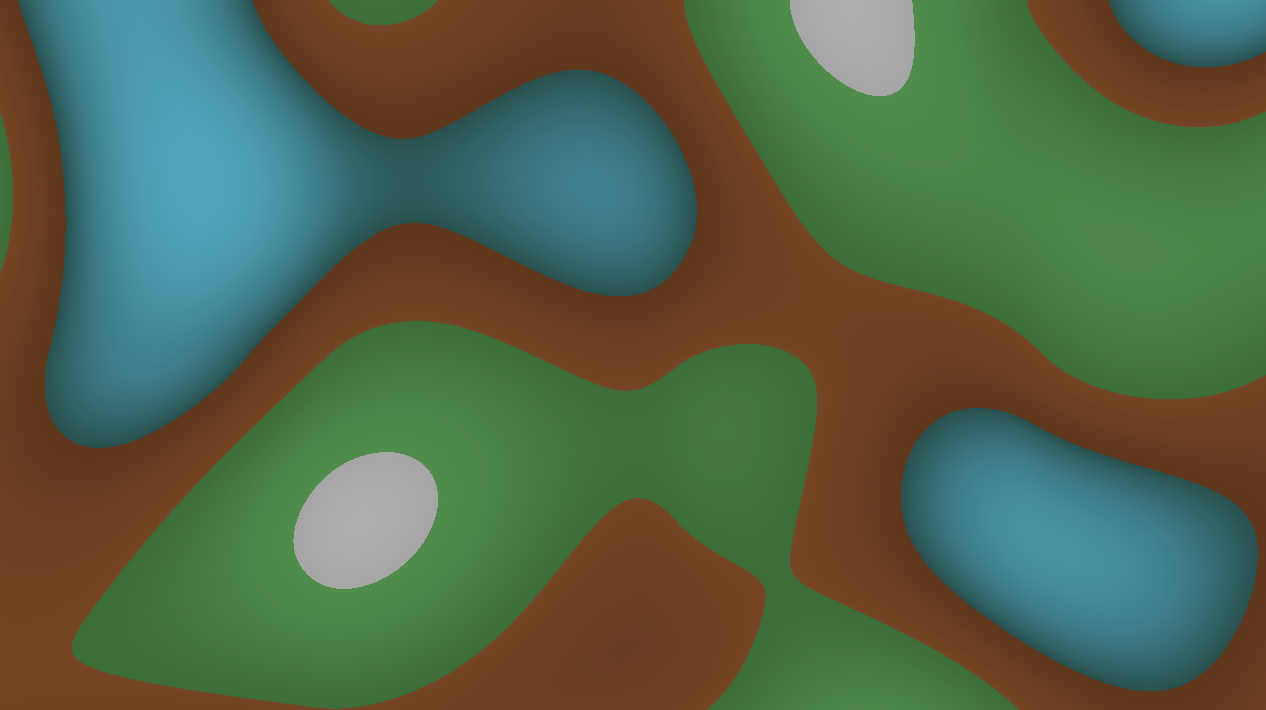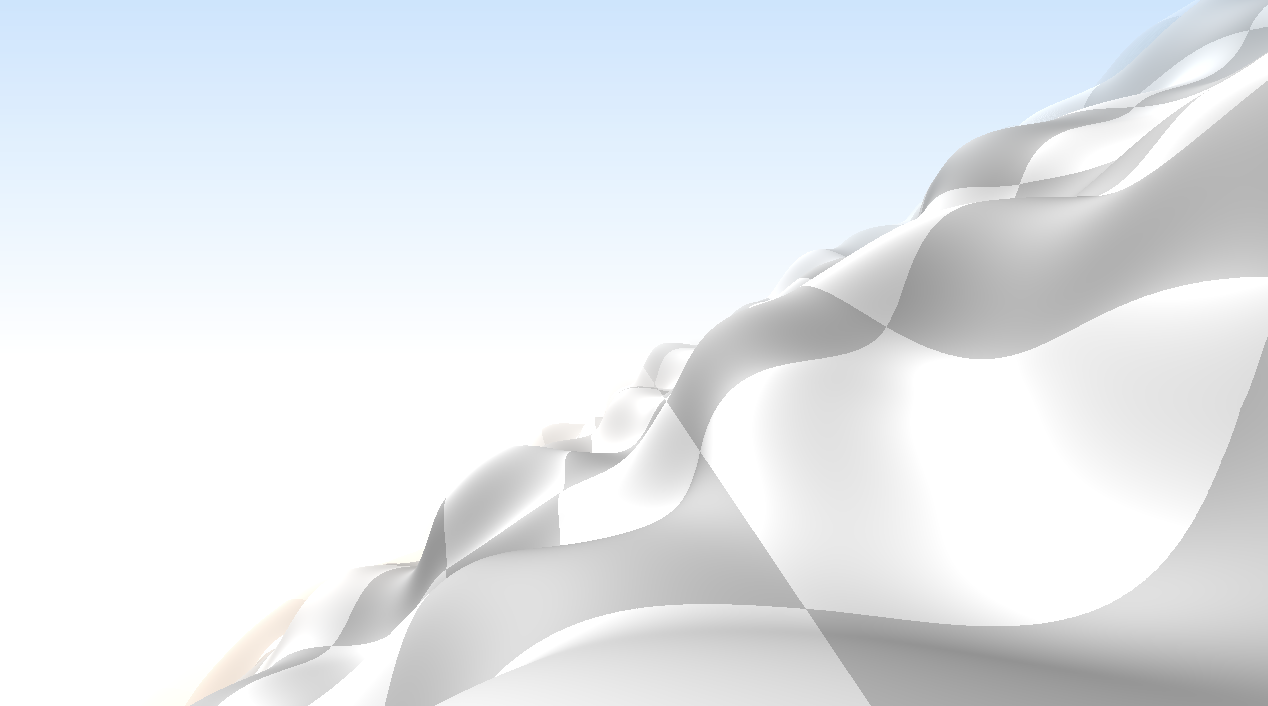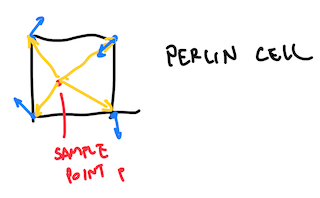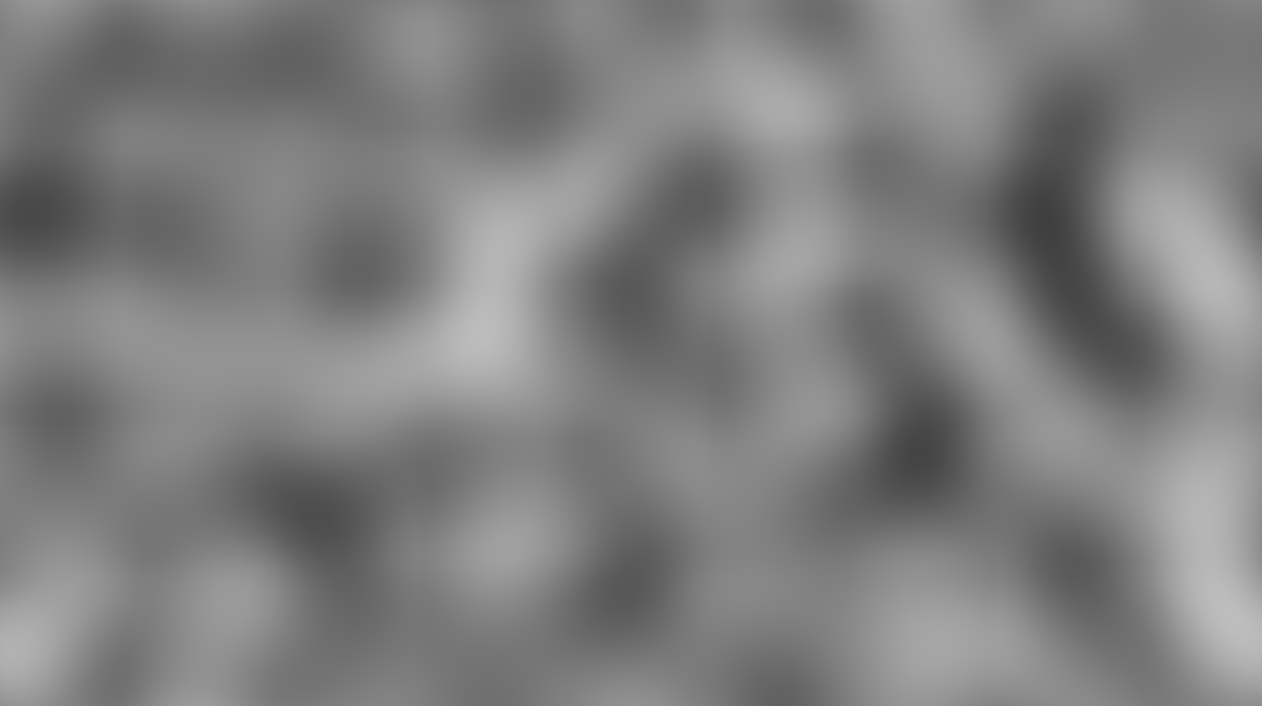A semi-curated blog of computer graphics and rendering.

## What is Perlin Noise?

To understand what Perlin noise is, we have to look at what regular noise and hash functions are first. Go on, give it a read. I will wait for you here. Alright. Have you read that? Cool. Now let’s proceed.

Proposed by Ken Perlin, Perlin noise is really similar to value noise. But instead of interpolating 1-dimensional noise values, Perlin noise instead interpolates the dot product of multi-dimensional noise values. In this way, it is much more chaotic (as a result, less blocky,) and the overall algorithm produces a smoother noise as a result.

Perlin noise is widely used in terrain generation, textures, and basically everything rendering related. It is used to add detail to a lot of textures. It’s the building block of a mathematical world. Take a look at this terrain generation shader (that I wrote):Or, this Perlin coaster ride, that I also wrote:Perlin noise is also an instrumental first step in generating Inigo Quilez’s Clouds shader’s volume. This is how that looks like:## 2D Hash Function

Since 2D Perlin noise interpolates 2-dimensional noise value instead of one, a vector hash function is needed. Not much is changed from the hash function, except this time it’s two dimensional.

vec2 rand2d(vec2 uv) {
return fract(sin(vec2(dot(uv, vec2(12.34, 45.67)),
dot(uv, vec2(78.9, 3.14)))) * 12345.67) * 2.0 - 1.0;
}


You can also write that as a matrix multiplication, as is the case of XorDev’s hash2d implementation. Once the 2D hash function is implemented, we can proceed to implement Perlin noise.

## Perlin Noise Implementation

The recipe is as follows:

1. Sample the four corners of the current cell (four 2-dimensional vectors)
2. Evaluate the dot products of the sampled result and the vector of sample point $$p$$ pointing towards the cell
3. Interpolate the four results using cubic interpolation (or quintic)If you are a little bit puzzled about what the hell step 2 is about, the four dot product are the yellow arrows dotting with their respective corner’s blue arrows. Step 3 is then executed to interpolate those dot products and make the end result look smooth. Here’s how it looks like in GLSL:

float perlin(vec2 uv) {
vec2 u = floor(uv);
vec2 f = fract(uv);
vec2 s = smoothstep(0.0, 1.0, f);

vec2 a = rand2d(u);
vec2 b = rand2d(u + vec2(1.0, 0.0));
vec2 c = rand2d(u + vec2(0.0, 1.0));
vec2 d = rand2d(u + vec2(1.0, 1.0));

return mix(mix(dot(a, -f), dot(b, vec2(1.0, 0.0) - f), s.x),
mix(dot(c, vec2(0.0, 1.0) - f), dot(d, vec2(1.0, 1.0) - f), s.x), s.y);
}


Since a dot product is involved, the end result of Perlin noise can be negative. In which case, a simple perlin(uv) * 0.5 + 0.5 should bring it back. Here’s how it it should look like: